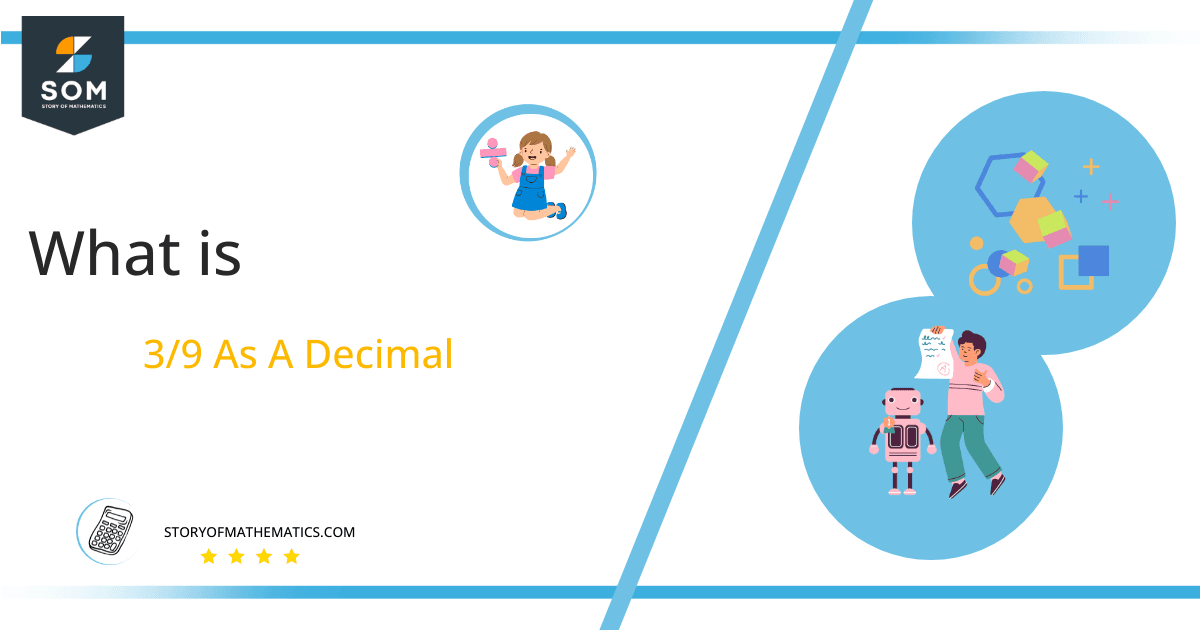# What Is 3/9 as a Decimal + Solution With Free Steps

The fraction 3/9 as a decimal is equal to 0.333.

A fraction can be converted into a decimal number by using the long division method. Expressing a number in the form of a decimal, fraction or percentage is a different way of exhibiting the same quantity. The division is one of the most used basic arithmetic operations which is used to convert a fraction into decimal form.Here, we are more interested in the division types that result in a Decimal value, as this can be expressed as a Fraction. We see fractions as a way of showing two numbers having the operation of Division between them that result in a value that lies between two Integers.

Now, we introduce the method used to solve said fraction to decimal conversion, called Long Division which we will discuss in detail moving forward. So, let’s go through the Solution of fraction 3/9. Given below is the division shown in figure 1:Figure 1

## Solution

First, we convert the fraction components i.e., the numerator and the denominator, and transform them into the division constituents i.e., the Dividend and the Divisor respectively.

This can be seen done as follows:

Dividend = 3

Divisor = 9

Now, we introduce the most important quantity in our process of division, this is the Quotient. The value represents the Solution to our division, and can be expressed as having the following relationship with the Division constituents:

Quotient = Dividend $\div$ Divisor = 3 $\div$ 9

This is when we go through the Long Division solution to our problem.

## 3/9 Long Division Method

We start solving a problem using the Long Division Method by first taking apart the division’s components and comparing them. As we have 3, and 9 we can see how 3 is Smaller than 9, and to solve this division we require that 3 be Bigger than 9.

This is done by multiplying the dividend by 10 and checking whether it is bigger than the divisor or not. If it is then we calculate the Multiple of the divisor which is closest to the dividend and subtract it from the Dividend. This produces the Remainder which we then use as the dividend later.

Now, we begin solving for our dividend 3, which after getting multiplied by 10 becomes 30.

We take this 30 and divide it by 9, this can be seen done as follows:

30 $\div$ 9 $\approx$ 3

Where:

9 x 3 = 27

This will lead to the generation of a Remainder equal to 30 – 27 = 3, now this means we have to repeat the process by Converting the 3 into 30 and solving for that:

30 $\div$ 9 $\approx$ 3

Where:

9 x 3 = 27

This, therefore, produces another remainder which is equal to 30 – 27 = 3. Now we must solve this problem to Third Decimal Place for accuracy, so we repeat the process with dividend 30.

30 $\div$ 9 $\approx$ 3

Where:

9 x 3 = 27

Finally, we have a Quotient generated after combining the three pieces of it as 0.333, with a Remainder equal to 3. It is a recurring decimal as the same number is repeated again and again therefore the decimal number is written in 3 decimal places.Images/mathematical drawings are created with GeoGebra.